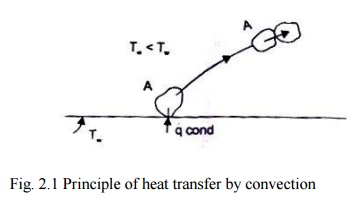Home | | Heat and Mass Transfer | Convection Heat Transfer

# Convection Heat Transfer

The heat transfer by convection requires a solid-fluid interface, a temperature difference between the solid surface and the surrounding fluid and a motion of the fluid.

CONVECTION

Convection Heat Transfer-Requirements

The heat transfer by convection requires a solid-fluid interface, a temperature difference between the solid surface and the surrounding fluid and a motion of the fluid. The process of heat transfer by convection would occur when there is a movement of macro-particles of the fluid in space from a region of higher temperature to lower temperature.

Convection Heat Transfer Mechanism

Let us imagine a heated solid surface, say a plane wall at a temperature Tw placed in an atmosphere at temperature T ¥, Fig. 2.1 Since all real fluids are viscous, the fluid particles adjacent to the solid surface will stick to the surface. The fluid particle at A, which is at a lower temperature, will receive heat energy from the plate by conduction. The internal energy of the particle would Increase and when the particle moves away from the solid surface (wall or plate) and collides with another fluid particle at B which is at the ambient temperature, it will transfer a part of its stored energy to B. And, the temperature of the fluid particle at B would increase. This way the heat energy is transferred from the heated plate to the surrounding fluid. Therefore the process of heat transfer by convection involves a combined action of heat conduction, energy storage and transfer of energy by mixing motion of fluid particles.Fig. 2.1 Principle of heat transfer by convection

Free and Forced Convection

When the mixing motion of the fluid particles is the result of the density difference caused by a temperature gradient, the process of heat transfer is called natural or free convection. When the mixing motion is created by an artificial means (by some external agent), the process of heat transfer is called forced convection Since the effectiveness of heat transfer by convection depends largely on the mixing motion of the fluid particles, it is essential to have a knowledge of the characteristics of fluid flow.

Basic Difference between Laminar and Turbulent Flow

In laminar or streamline flow, the fluid particles move in layers such that each fluid p article follows a smooth and continuous path. There is no macroscopic mixing of fluid particles between successive layers, and the order is maintained even when there is a turn around a comer or an obstacle is to be crossed. If a lime dependent fluctuating motion is observed indirections which are parallel and transverse to t he main flow, i.e., there is a radom macroscopic mixing of fluid particles across successive layers of fluid flow, the motion of the fluid is called' turbulent flow'. The path of a fluid particle would then be zigzag and irregular, but on a statistical basis, the overall motion of the macroparticles would be regular and predictable.

Study Material, Lecturing Notes, Assignment, Reference, Wiki description explanation, brief detail
Mechanical : Heat and Mass Transfer : Conduction : Convection Heat Transfer |